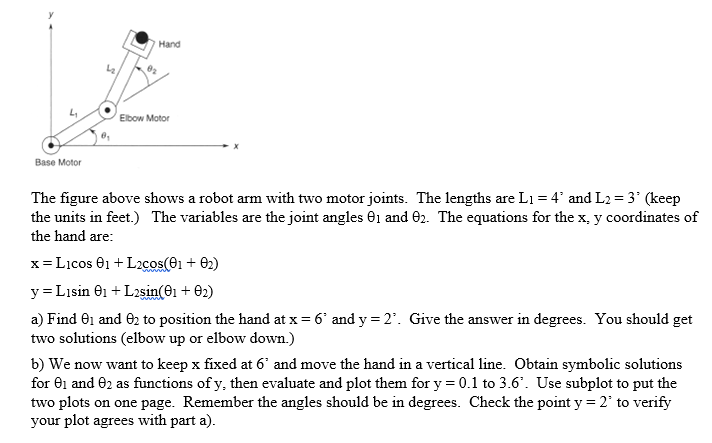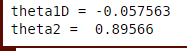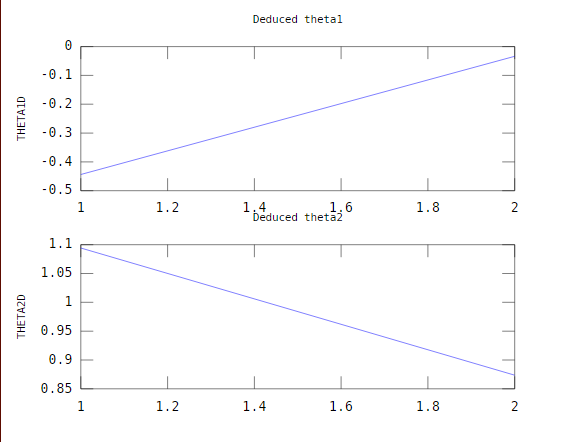# Question & Answer: Use MATLAB to do this problem The figure above shows a robot arm with two motor joints. The lengths are L_1 = 4' and L_2 = 3' (keep the units in…..

Use MATLAB to do this problem

The figure above shows a robot arm with two motor joints. The lengths are L_1 = 4′ and L_2 = 3′ (keep the units in feet.) The variables are the joint angles theta_1 and theta_2. The equations for the x, y coordinates of the hand are: x = L_1 cos theta_1 + L_2 cos (theta_1 + theta_2) y = L_1 sin theta_1 + L_2 sin (theta_1 + theta_2) a) Find theta_1 and theta_2 to position the hand at x = 6 and y = 2. Give the answer in degrees. You should get two solutions (elbow up or elbow down.) b) We now want to keep x fixed at 6′ and move the hand in a vertical line. Obtain symbolic solutions for theta_1 and theta_2 as functions of y, then evaluate and plot them for y = 0.1 to 3.6′. Use subplot to put the two plots on one page. Remember the angles should be in degrees. Check the point y = 2 to verify your plot agrees with part a).Don't use plagiarized sources. Get Your Custom Essay on
Question & Answer: Use MATLAB to do this problem The figure above shows a robot arm with two motor joints. The lengths are L_1 = 4' and L_2 = 3' (keep the units in…..
GET AN ESSAY WRITTEN FOR YOU FROM AS LOW AS \$13/PAGE

Here is the solution of the given algorithm on the basis of given criteria:-
```L1=4;  % length of first arm
L2=3;  % length of second arm
x=6; % given values of x co-ordinates
```
```y=2; % given values of y co-ordinates
```
```%* The values of theta1 and theta2 are deduced mathematically from the x and y coordinates  by using inverse kinematics formulae. *%
c2 = (x.^2 + y.^2 - L1^2 - L2^2)/(2*L1*L2);
s2 = sqrt(1 - c2.^2);
theta2=atan2(s2,c2); % theta2 is deduced in degrees
```
```k1 = L1 + L2.*c2;
k2 = L2*s2;
theta1D = atan2(y,x) - atan2(k2,k1); % theta1 is deduced in degrees
```

theta1D

theta2

Please run these commands into your MATLAB window and check out the output.

Here is the output shown below:-=========================================================

Part B:-

L1=4; % length of first arm
L2=3; % length of second arm
x=6; % fixed values of x co-ordinates
y=0.1:2:3.6; % given values of y co-ordinates
%* The values of theta1 and theta2 are deduced mathematically from the x and y coordinates by using inverse kinematics formulae. *%
c2 = (x.^2 + y.^2 – L1^2 – L2^2)/(2*L1*L2);
s2 = sqrt(1 – c2.^2);
theta2=atan2(s2,c2); % theta2 is deduced in degrees

k1 = L1 + L2.*c2;
k2 = L2*s2;
theta1D = atan2(y,x) – atan2(k2,k1);
% theta1 is deduced in degrees
subplot(2,1,1);
plot(theta1D);
ylabel(‘THETA1D’,’fontsize’,8)
title(‘Deduced theta1′,’fontsize’,8)

subplot(2,1,2);
plot(theta2);
ylabel(‘THETA2D’,’fontsize’,8)
title(‘Deduced theta2′,’fontsize’,8)

Here is the output shown below:-